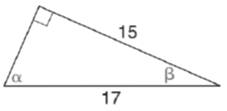Chapter 11.3, Problem 2E### Elementary Geometry for College St...

6th Edition
Daniel C. Alexander + 1 other
ISBN: 9781285195698

#### Solutions

Chapter
Section### Elementary Geometry for College St...

6th Edition
Daniel C. Alexander + 1 other
ISBN: 9781285195698
Textbook Problem
1 views

# In Exercises 1 to 4, find tan α and tan β for each triangle.To determine

To find:

The values of tanα and tanβ for the triangle.

Explanation

Given:

The triangle is shown below.

Definition used:

In a right triangle, the tangent ratio for an acute angle is the ratio oppositeadjacent.

Calculation:

Let x be the unknown side in the given figure.

(17)2=x2+152289=x2

### Still sussing out bartleby?

Check out a sample textbook solution.

See a sample solution

#### The Solution to Your Study Problems

Bartleby provides explanations to thousands of textbook problems written by our experts, many with advanced degrees!

Get Started

#### Find more solutions based on key concepts